Include your answers in this document in the sections below the rubric where I have point values indicated (1 p).

# Rubric

Answer the questions with the data examples.

# Paired Comparisons of Two Sleep Remedies

The following data give the amount of sleep gained in hours from two sleep remedies, A and B, applied to 10 individuals who have trouble sleeping an adequate amount. Negative values imply sleep loss.

Let $$\mu_A$$ = population mean sleep gain (among troubled sleepers) on remedy A, and $$\mu_B$$ = population mean sleep gain (among troubled sleepers) on remedy B. Consider testing $$H_0: \mu_B - \mu_A = 0$$ or equivalently $$\mu_D = 0$$, where $$\mu_D = \mu_B - \mu_A$$.

library(tidyverse)
## -- Attaching packages ---------------------------------------------------------------------------------------- tidyverse 1.2.1 --
## v ggplot2 3.2.1     v purrr   0.3.2
## v tibble  2.1.3     v dplyr   0.8.3
## v tidyr   1.0.0     v stringr 1.4.0
## v readr   1.3.1     v forcats 0.4.0
## -- Conflicts ------------------------------------------------------------------------------------------- tidyverse_conflicts() --
## x dplyr::lag()    masks stats::lag()
# Data
A    B
0.7  1.9
-1.6  0.8
-0.2  1.1
-1.2  0.1
0.1 -0.1
3.4  4.4
3.7  5.5
0.8  1.6
0.0  4.6
2.0  3.0

# calculate paired difference bewteen two remedies, D = B - A
dat_sleep <-
dat_sleep %>%
mutate(
D = B - A
)
summary(dat_sleep)
##        A               B                D
##  Min.   :-1.60   Min.   :-0.100   Min.   :-0.200
##  1st Qu.:-0.15   1st Qu.: 0.875   1st Qu.: 1.000
##  Median : 0.40   Median : 1.750   Median : 1.250
##  Mean   : 0.77   Mean   : 2.290   Mean   : 1.520
##  3rd Qu.: 1.70   3rd Qu.: 4.050   3rd Qu.: 1.675
##  Max.   : 3.70   Max.   : 5.500   Max.   : 4.600

A plot of both sleep remedies, with a 1-to-1 reference line to indicate which remedy is better for which people.

# determine range of each axis to use most extreme of each for square plot below
axis.lim <- range(c(dat_sleep$A, dat_sleep$B))

library(ggplot2)
# scatterplot of A and B sleep times, with 1:1 line
p <- ggplot(dat_sleep, aes(x = A, y = B))
# draw a 1:1 line, dots above line indicate "B > A"
p <- p + geom_abline(intercept = 0, slope = 1, alpha = 0.4)
p <- p + geom_vline(xintercept = 0, alpha = 0.2)
p <- p + geom_hline(yintercept = 0, alpha = 0.2)
p <- p + geom_point()
p <- p + geom_rug()
# make the axes square so it's a fair visual comparison
p <- p + coord_equal()
p <- p + scale_x_continuous(limits=axis.lim)
p <- p + scale_y_continuous(limits=axis.lim)
p <- p + labs(title = "Sleep hours gained on two sleep remedies: A vs B")
print(p)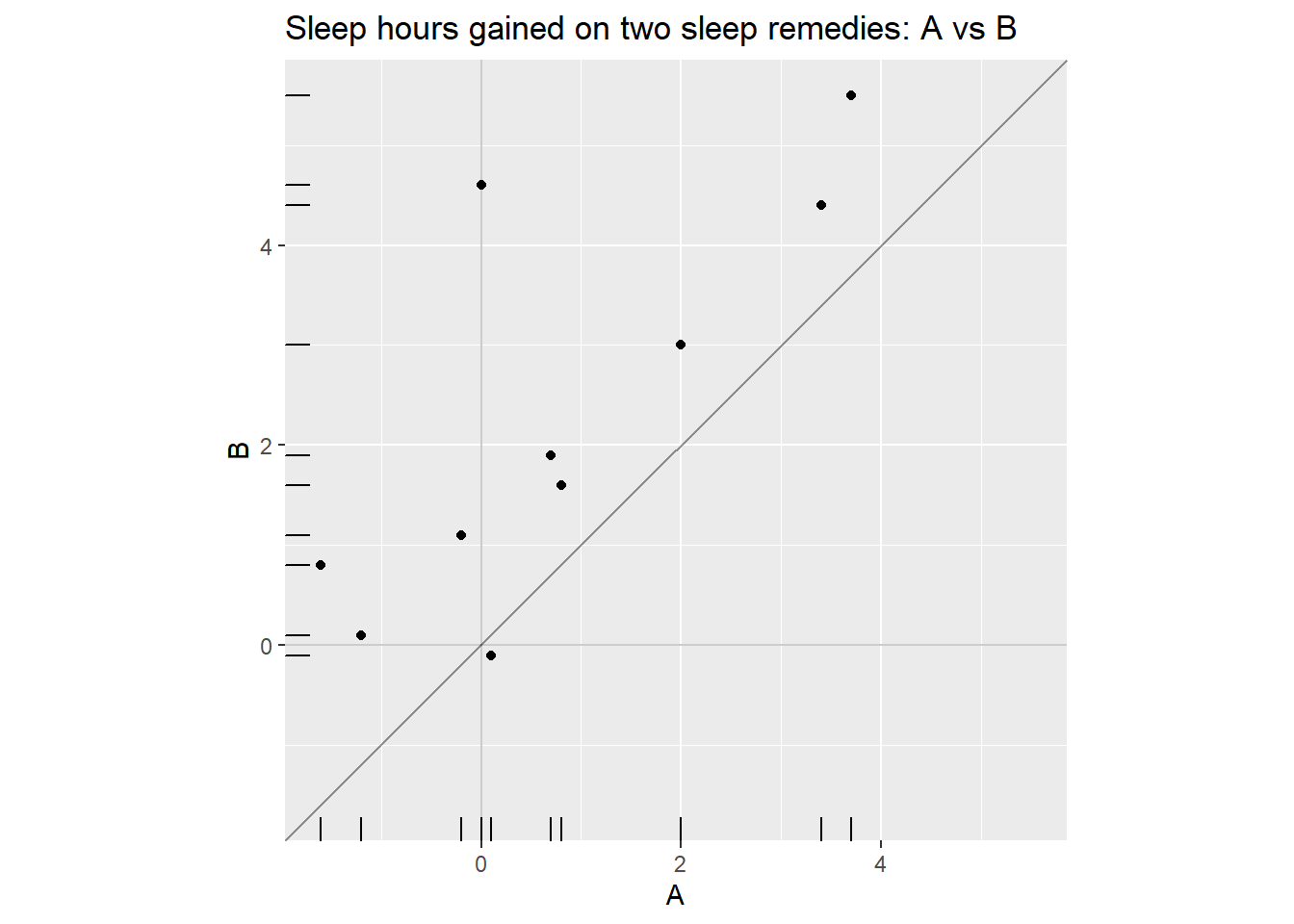A plot of the paired differences.

library(ggplot2)
p1 <- ggplot(dat_sleep, aes(x = D))
p1 <- p1 + scale_x_continuous(limits=c(-5,+5), breaks = seq(-6, 6, by=2))
p1 <- p1 + geom_histogram(aes(y=..density..), binwidth = 0.5)
p1 <- p1 + geom_density(alpha=0.1, fill="white", adjust = 2)
# vertical reference line at 0
p1 <- p1 + geom_vline(xintercept = 0, colour="red", linetype="dashed")
p1 <- p1 + geom_vline(xintercept = mean(dat_sleep$D), colour="blue", alpha = 0.5) p1 <- p1 + geom_rug() p1 <- p1 + labs(title = "Difference of sleep hours gained: D = B - A") print(p1) ## Warning: Removed 2 rows containing missing values (geom_bar).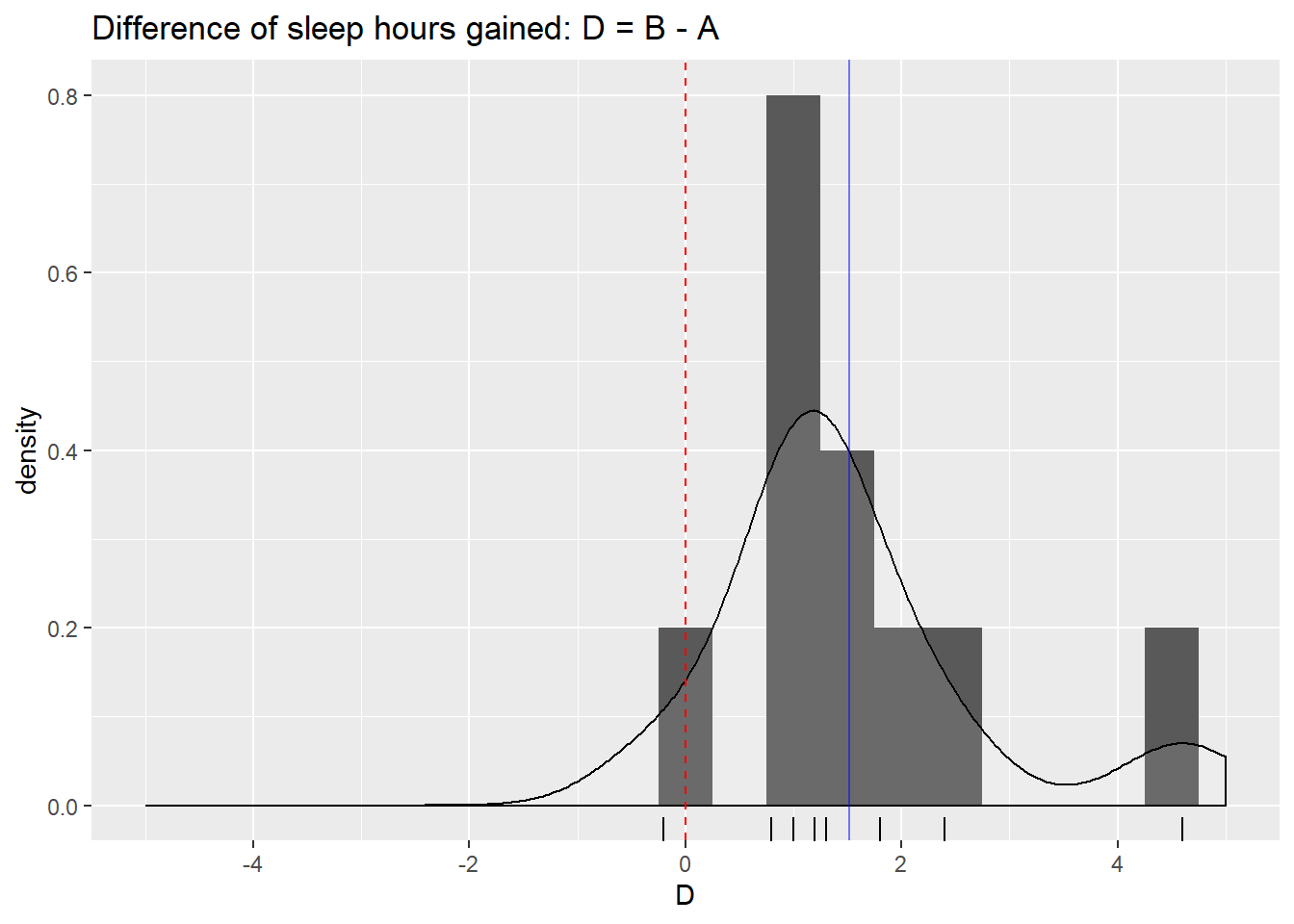Hypothesis test 1. (2 p) ’’ •$H_0: $versus$H_A: $2. Let $$\alpha = 0.05$$, the significance level of the test and the Type-I error probability if the null hypothesis is true. # one-sample t-test of differences (paired t-test) # t.summary <- t.test(dat_sleep$D)  # this gives the same result
t.summary <- t.test(dat_sleep$B, dat_sleep$A, paired = TRUE)
t.summary
##
##  Paired t-test
##
## data:  dat_sleep$B and dat_sleep$A
## t = 3.7796, df = 9, p-value = 0.004352
## alternative hypothesis: true difference in means is not equal to 0
## 95 percent confidence interval:
##  0.610249 2.429751
## sample estimates:
## mean of the differences
##                    1.52
1. (1 p) $$t_{s} = 99$$.

2. (1 p) $$p = 99$$, this is the observed significance of the test.

3. (2 p) Because $$p = 99$$, …

# Test assumptions

For one- and two-sample $$t$$-tests, our assumptions are that we have a representative (random) sample from the population (not something that can be tested very well), the the sampling distributions of our test statistic $$t$$ is normal.

Hidden in a code chunk are functions for one-sample and two-sample boostrap appoximations of the sampling distributions of the mean to compare with a normal distribution for the normality assumption.

## Paired Comparisons of Two Sleep Remedies

1. Check assumptions of the test.

Description of plots: The top plot is of the data, a histogram with smoothed density curve. The bottom plot is a comparison of the boostrap appoximation of the sampling distributions of the mean. If the histogram and smooth density curve are very close (basically underneath) the red normal distribution, then we can say the sampling distribution is consistent with normality. If there is systematic bias (as below) where one side is over and the other side is under the red curve, then that’s evidence against normality, in which case we would pursue a nonparametric test (next week).

bs.one.samp.dist(dat_sleep$D)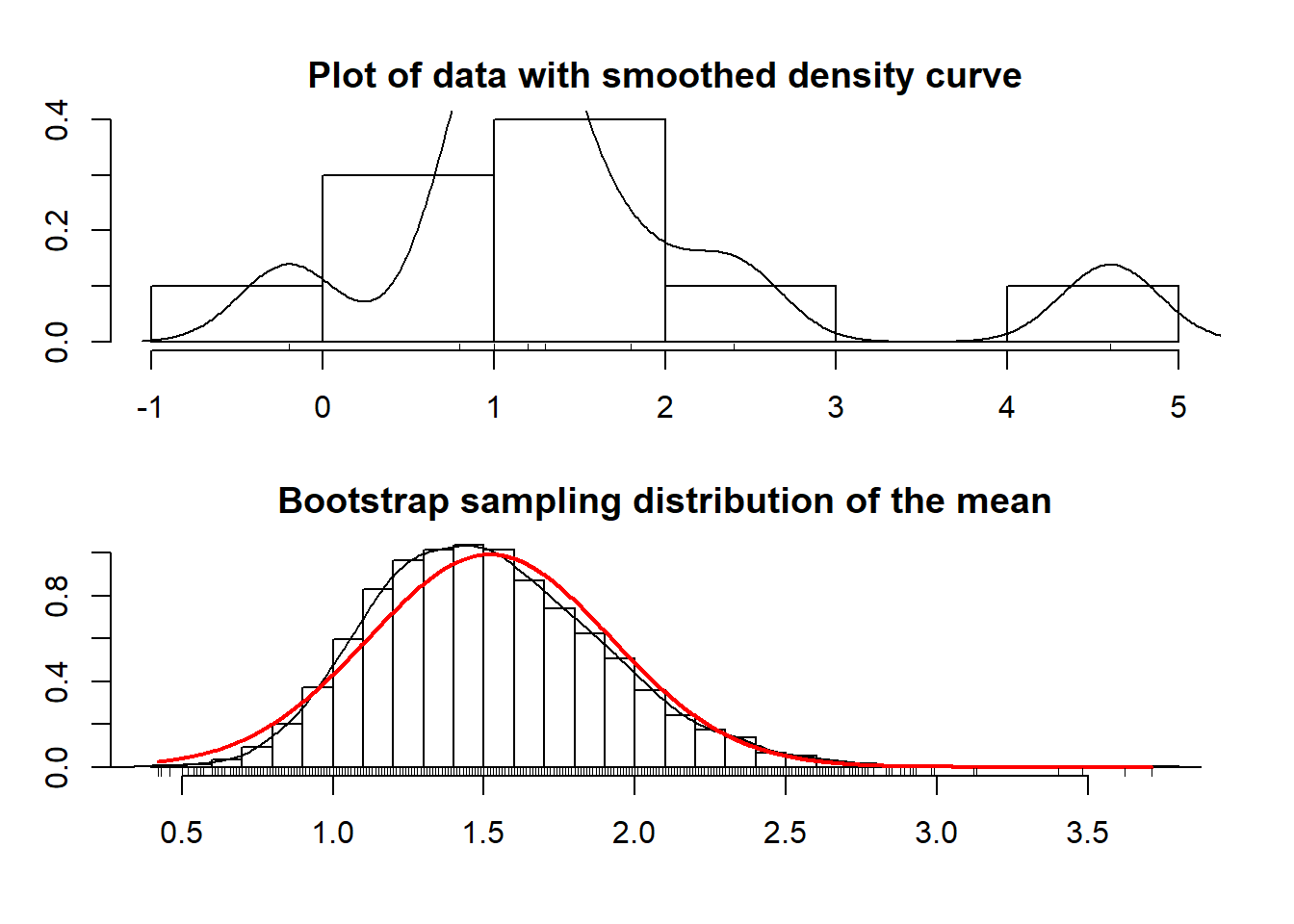(2 p) Interpret the plots above. # African countries in the UN example Check the assumptions of the two-sample $$t$$-test for this example (explained in a previous worksheet). # install.packages("gsheet") library(gsheet) dat_UN_Africa_url <- "docs.google.com/spreadsheets/d/1PkjIygZdtlopH6kvyle-KeoECsycHWyCaya92erlvaE" dat_UN_Africa <- gsheet2tbl(dat_UN_Africa_url) %>% as.data.frame() %>% na.omit() %>% mutate( PrimingNumber = factor(PrimingNumber) , HighLow = factor(HighLow) ) %>% filter( Class == "F19" ) str(dat_UN_Africa) ## 'data.frame': 69 obs. of 4 variables: ##$ PrimingNumber: Factor w/ 2 levels "10","65": 2 2 2 2 1 1 1 1 1 2 ...
##  $HighLow : Factor w/ 2 levels "H","L": 2 2 2 2 1 1 1 1 1 2 ... ##$ UN_Percentage: num  3 25 30 34 28 15 26 14 35 20 ...
##  $Class : chr "F19" "F19" "F19" "F19" ... Here are some summaries and plots. ## If we create a summary data.frame with a similar structure as our data, then we ## can annotate our plot with those summaries. # calculate the estimated mean and order M then F est_mean <- as.numeric(by(dat_UN_Africa$UN_Percentage, dat_UN_Africa$PrimingNumber, mean)) # combine true US mean with our estimated mean mean_UN_Africa <- data.frame(PrimingNumber = rev(unique(dat_UN_Africa$PrimingNumber))
, UN_Percentage = est_mean)
mean_UN_Africa <-
mean_UN_Africa %>%
mutate(
PrimingNumber = factor(PrimingNumber)
)
mean_UN_Africa
##   PrimingNumber UN_Percentage
## 1            10      17.97222
## 2            65      28.15152
# summary
by(dat_UN_Africa$UN_Percentage, dat_UN_Africa$PrimingNumber, summary)
## dat_UN_Africa$PrimingNumber: 10 ## Min. 1st Qu. Median Mean 3rd Qu. Max. ## 2.00 12.00 16.00 17.97 25.00 38.00 ## -------------------------------------------------------- ## dat_UN_Africa$PrimingNumber: 65
##    Min. 1st Qu.  Median    Mean 3rd Qu.    Max.
##    3.00   17.00   25.00   28.15   35.00   83.00
# histogram using ggplot
p <- ggplot(dat_UN_Africa, aes(x = UN_Percentage))
p <- p + geom_histogram(binwidth = 4)
p <- p + geom_rug()
p <- p + geom_vline(data = mean_UN_Africa, aes(xintercept = UN_Percentage), colour = "red")
p <- p + facet_grid(PrimingNumber ~ .)
print(p)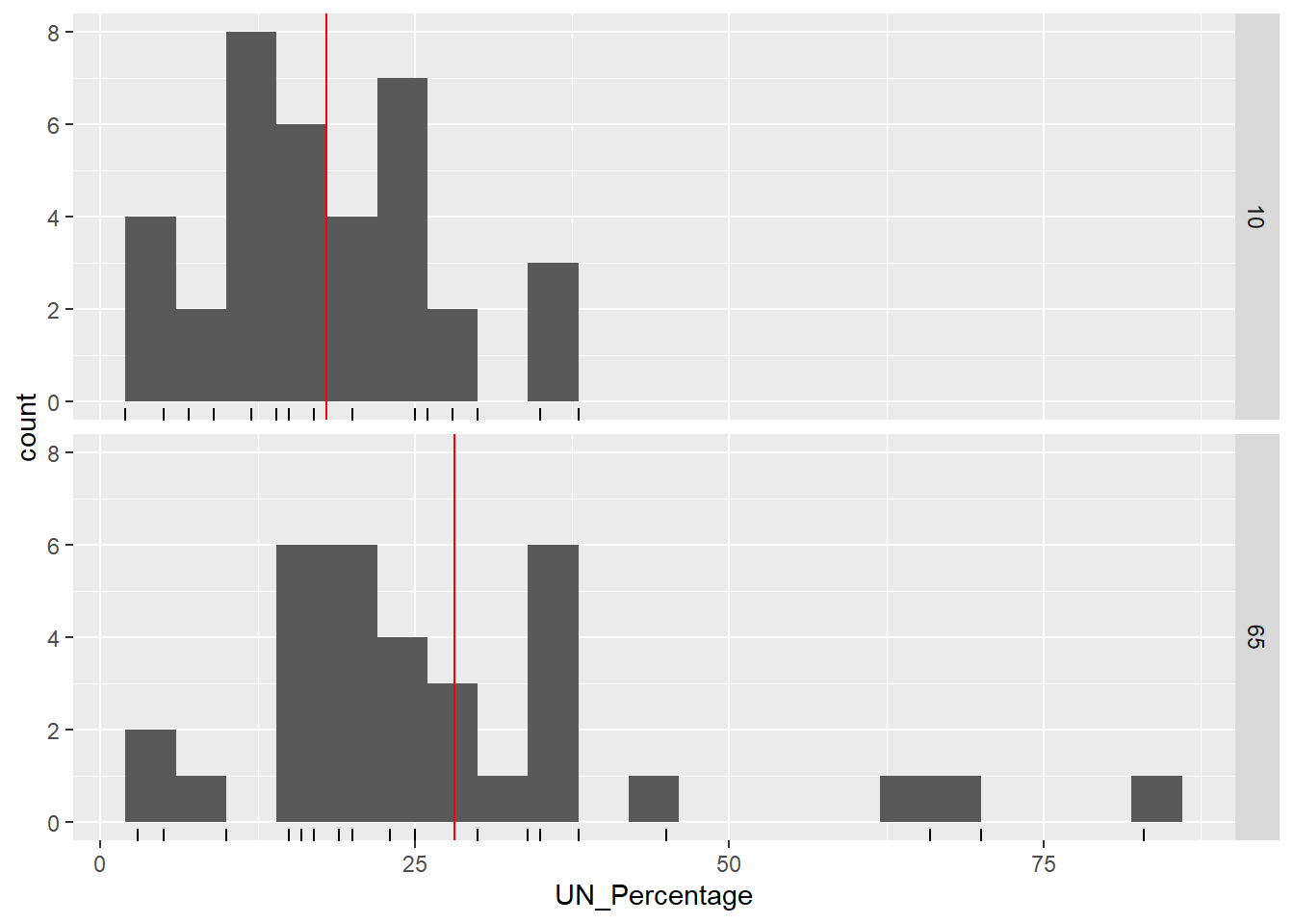A priori, before we observed the data, we hypothesized that those who were primed with a larger number (65) would provide a higher percentage (UN_Percentage) than those with the lower number (10). Therefore, this is a one-sided test.

# two-sample t-test
t_summary_UN <- t.test(UN_Percentage ~ PrimingNumber, data = dat_UN_Africa
, alternative = "less")
t_summary_UN
##
##  Welch Two Sample t-test
##
## data:  UN_Percentage by PrimingNumber
## t = -2.9877, df = 47.438, p-value = 0.00222
## alternative hypothesis: true difference in means is less than 0
## 95 percent confidence interval:
##       -Inf -4.463638
## sample estimates:
## mean in group 10 mean in group 65
##         17.97222         28.15152
1. Check assumptions of the test.
bs.two.samp.diff.dist(subset(dat_UN_Africa, PrimingNumber == "10")$UN_Percentage , subset(dat_UN_Africa, PrimingNumber == "65")$UN_Percentage)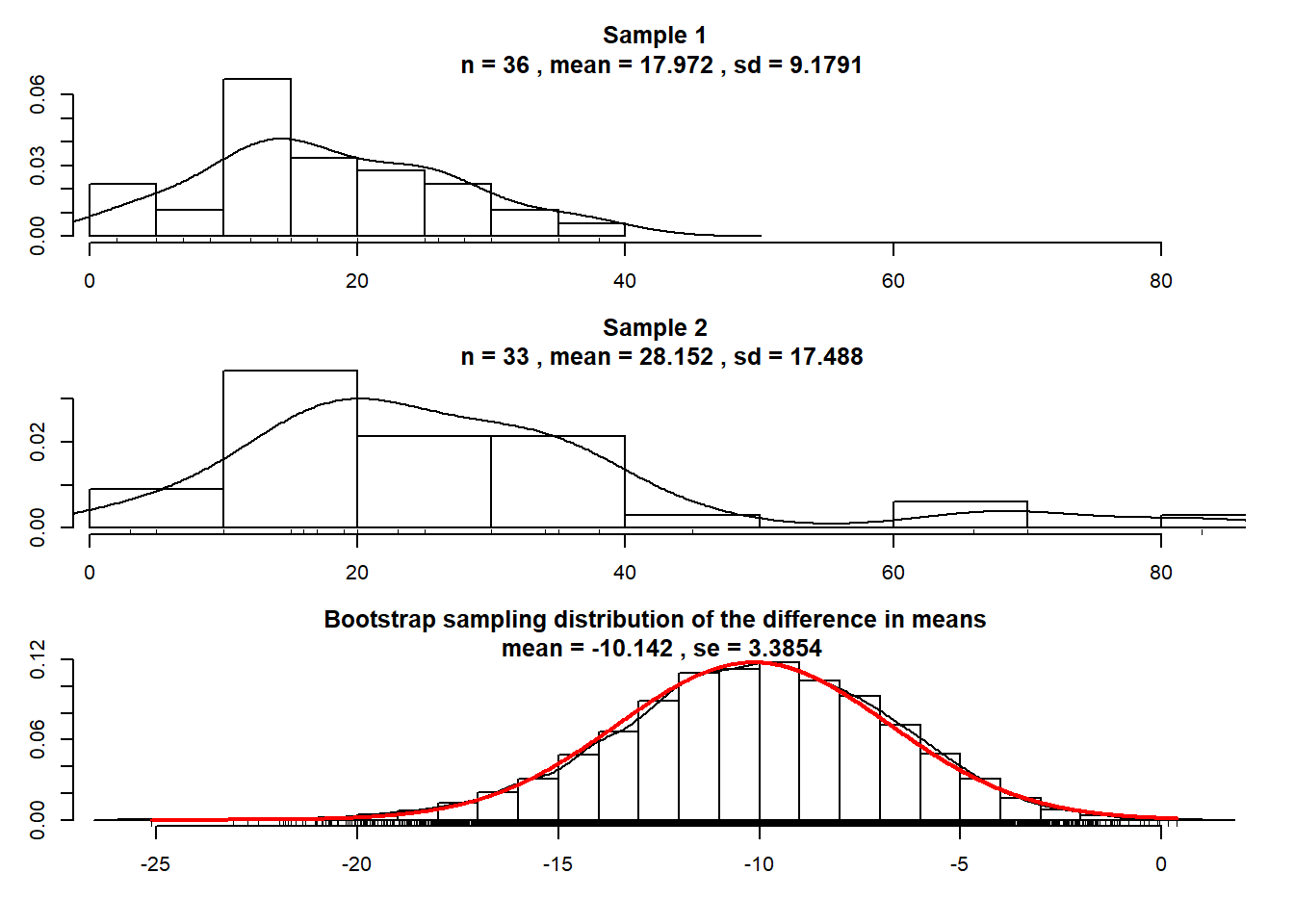(2 p) Interpret the plots above.